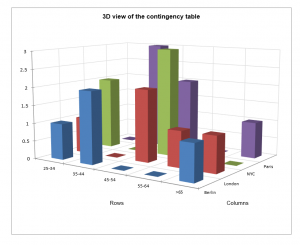# Contingency table (descriptive statistics)

Contingency tables let you visualize the relationship between two qualitative variables. Describe contingency tables in Excel using the XLSTAT software.This tool allows you to compute a variety of descriptive statistics on a contingency table. A chi-square test is optionally performed. Additional tests on contingency tables are available in the Tests on contingency tables feature.

## What is a contingency table?

A contingency table, also called a cross-tab, is an efficient way to summarize the relation (or correspondence) between two categorical variables V1 and V2. It has the following structure:

 V1 V2 Category 1 Category j … Category m2 Category 1 n(1,1) n(1,j) … n(1,m2) … … … … … Category i n(i,1) n(i,j) … n(i,m2) … … … … … Category m1 n(m1,1) n(m1,j) … n(m1,m2)

where n(i,j) is the frequency of observations that show both characteristic i for variable V1, and characteristic j for variable V2.

## Options for Contingency tables in XLSTAT

### Criteria to characterize the link between the two qualitative variables

XLSTAT provides you with two criteria to characterize the relationship between the two variables:

• The Chi-square distance has been suggested to measure the distance between two categories. The Pearson chi-square statistic, which is the sum of the Chi-square distances, is used to test the independence between rows and columns.
• Inertia is a measure inspired from physics that is often used in Correspondence Analysis, a method that is used to analyse in depth contingency tables. The inertia of a set of points is the weighted mean of the squared distances to the center of gravity.

Many more indexes and tests are available in the Correlation and association tests feature to characterize the relationship between two categorical variables.

### Bootsrap confidence intervals

XLSTAT allows you to obtain bootstrap confidence interval around the theoretical frequency of each pair of categories in a contingency table. It offers an alternative to the classical Chi-square by cell.

The method is as follows:

1. Build a dataset with two qualitative variables using the value of the contingency table.

2. Randomly draw with replacement observations from the dataset for both variables independently.

3. Build a contingency table with the new dataset.

4. Repeat 2 and 3 as many times as specified by the user.

5. Compute mean, standard error, confidence interval and percentile confidence intervals for each pair of categories.

Pairs with observed value out of the confidence interval show a significant difference between the two categories (Amiri et al. 2011).

## Results for Contingency tables in XLSTAT

The following descriptive statistics are available within this tool:

• Inertia by cell.
• Chi-square by cell
• Significance by cell
• Observed frequencies
• Theoretical frequencies
• Proportions / row
• Proportions / column
• Proportions / total

The following graphs are available within this tool:

3D view of the contingency table: Activate this option to display the 3D bar chart corresponding to the contingency table.

Bar charts

• Grouped: Choose this option to display the graphs as bars grouped by modality.
• Stacked bars: Choose this option to display the chart as stacked bars. These charts are used to compare the frequencies of sub-samples to those of a full sample.

Check out our tutorial on contingency tables!### analyze your data with xlstat

14-day free trial

Included in

Related features